## Introduction

Linear regression is considered the most accurate method in segregating costs into Fixed and Variable components. Like the High-Low Method and other methods, the Least-Squares Method follows the same simple linear cost function:However, most people consider the Least-Squares Method more accurate, as it computes Fixed and Variable Costs mathematically. When looking into costs, we can present the formula like this: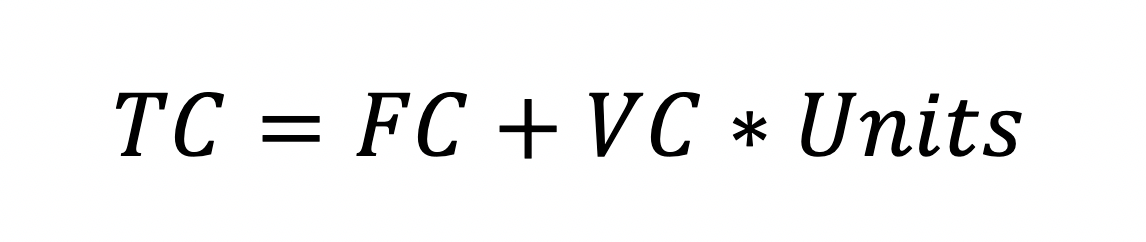Where:

• TC is Total Mixed Costs;
• FC represents the Fixed Costs that do not change depending on activity unit volume changes;
• VC is the variable cost associated with each additional unit of activity;
• Units are the units of measurement for the activity.

## Least-Squares Regression

The Least-Squares regression model is a statistical technique that may be used to estimate a linear total cost function for a mixed cost, based on past cost data. The function can then be used to forecast costs at different activity levels, as part of the budgeting process or to support decision-making processes.

Least-Squares Regression calculates a line of best fit to a set of data pairs, i.e., a series of activity levels and corresponding total costs.

The idea behind the calculation is to minimize the sum of the squares of the vertical distances (errors) between data points and the cost function.

In statistics, the lower error means better explanatory power of the regression model. The Least Squares model aims to define the line that minimizes the sum of the squared errors. We are trying to determine the line that is closest to all observations at the same time.

We need to be careful with outliers when applying the Least-Squares method, as it is sensitive to strange values pulling the line towards them. This is because the technique uses the squares of the variables, which increases the impact of outliers.

## How It Works

Looking into differential calculus, we get the following normal equations:Using the normal equations and the process of elimination we can derive a formula for b. We use this formula to calculate the Variable Costs when we apply the Least-Squares Method: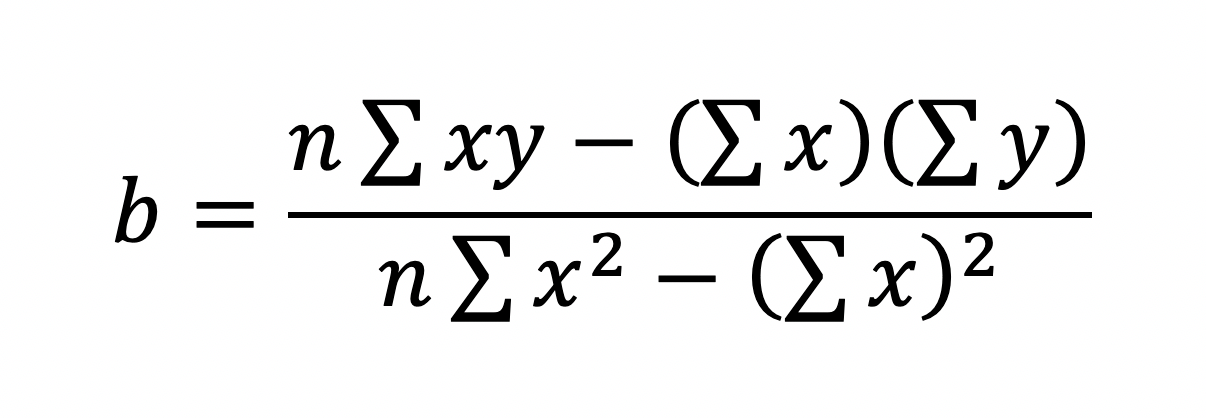After calculating the Variable Costs (b) per unit, we can then compute the Fixed Costs via the formula:Notice the accents above y and x. We use the means of the two variables. To calculate those we sum the variables in all observable data points and divide them by the number of data points, or we derive a simple average:Changing the means in the formula above with those formulas, we get an extended formula for the Fixed Costs:If we look at a graphical representation of the linear cost function, a is what we call the y-intercept of the line and equals the approximate Fixed Costs at any activity level, and b is the slope of the line and represents the Variable Costs per unit.## Example

Let us take a look at an example to see the Least-Squares Regression Model in action.

We have the following data on the costs for producing the last ten batches of a product. The data points show us the unit volume of each batch and the corresponding production costs.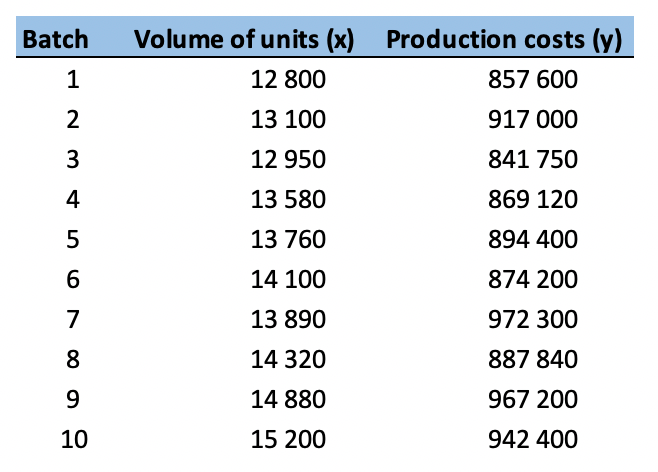Next, we can plot the data on a scatter plot to see if it looks linear.As the data seems a bit dispersed, let us calculate it’s correlation. We get a 0.64 correlation coefficient between volume of units and cost of production. Usually we consider values between 0.5 and 0.7 to represent a moderate correlation.To calculate the regression formulas we discussed, we need to add two help columns and calculate x * y and x2 for each batch. We also need the means for x (volume of units) and y (production costs).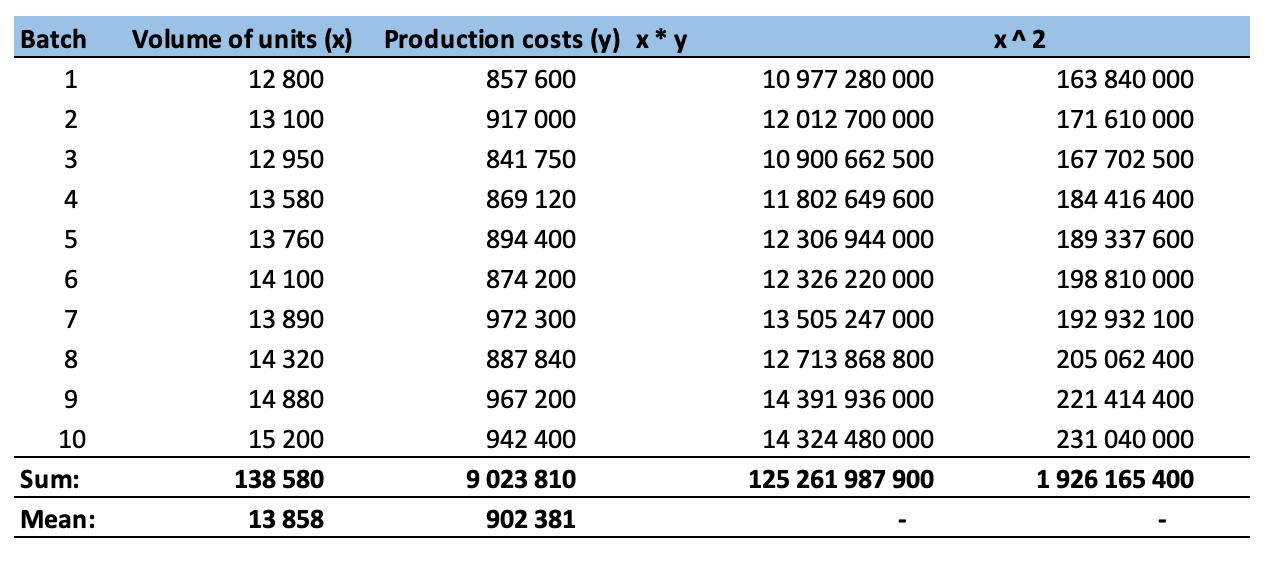After we have calculated the supporting values, we can go ahead and calculate our b. It represents the variable costs in our cost model and is called a slope in statistics.Having calculated the b of our model, we can go ahead and calculate the a. It represents the fixed costs and is called the y-intercept.We build the model function from the calculated y-intercept and slope of the function.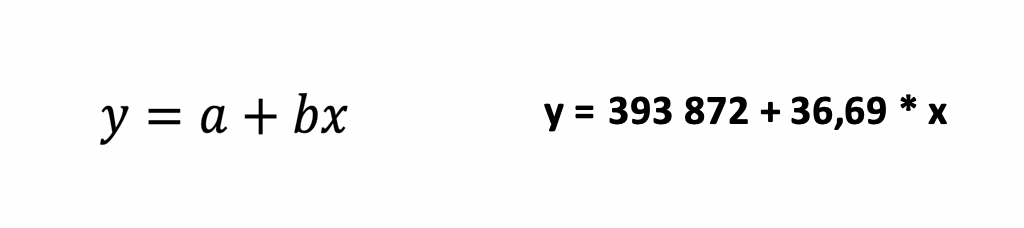The Least-Squares Method has some advantages and disadvantages that make it more desirable in certain situations:

(+) Simplicity – the method is easy to understand and perform;

(+) It’s applicable in almost any situation – honestly, it’s hard to think of a case where the Least-Squares method will be inapplicable;

(+) The technique has a strong underlying theoretical foundation in statistics;

() As we already noted, the method is susceptible to outliers, since the distance between data points and the cost function line are squared.

() It has an inherent assumption that the two analyzed variables have at least some kind of correlation.

() The Least-Squares method might yield unreliable results when the data is not normally distributed. However, this can be mitigated by including more data points in our sample.

## Conclusion

The Least Squares Method is probably one of the most popular predictive analysis techniques in statistics. It is widely used to fit a function to a data set. The simplest example is defining a straight-line, as we looked above, but this function can be a curve or even a hyper-surface in multivariate statistical analysis.

Professionals apply the method in a variety of fields like Medicine, Biology, Finance, Agriculture, Sociology, and others.## FCCA, FMVA

Hi! I am a finance professional with 10+ years of experience in audit, controlling, reporting, financial analysis and modeling. I am excited to delve deep into specifics of various industries, where I can identify the best solutions for clients I work with.

In my spare time, I am into skiing, hiking and running. I am also active on Instagram and YouTube, where I try different ways to express my creative side.

### 4 Responses

1. Ochulu edokache says:

I found this piece usefully

1. Dobromir Dikov says: